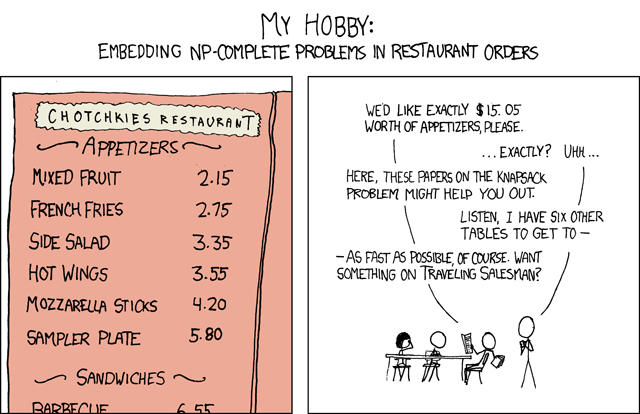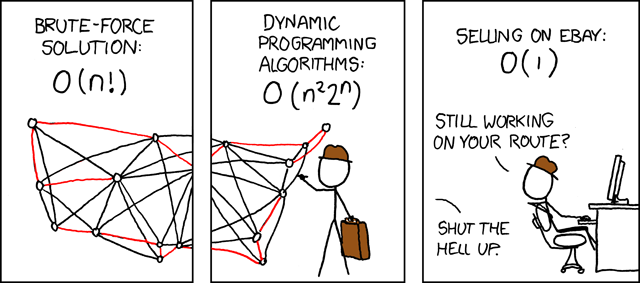# Welcome!

Our article was written using a component-based library called Idyll. In addition to buttons and sliders you will see the following in this article...

This component is an external link which will redirect you to another page.
This component is an internal link which will send you to a part of the page when clicked on.
This component is an action link which will trigger some event on the page to do something.

Let's Get Started!

# Introduction

Variations of the Traveling Salesman Problem (TSP) have existed since the 1800s. Generally speaking, the problem can be stated as:

Given a list of cities and the distances between each pair of cities, what is the shortest possible route that visits each city exactly once and returns to the origin city?

In essence, this question is asking us (the salesman) to visit each of the cities via the shortest path that gets us back to our origin city. We can conceptualize the TSP as a graph where each city is a node, each node has an edge to every other node, and each edge weight is the distance between those two nodes.

The first computer coded solution of TSP by Dantzig, Fulkerson, and Johnson came in the mid 1950’s with a total of 49 cities. Since then, there have been many algorithmic iterations and 50 years later, the TSP problem has been successfully solved with a node size of 24,978 cities! Multiple variations on the problem have been developed as well, such as mTSP, a generalized version of the problem and Metric TSP, a subcase of the problem.

The original Traveling Salesman Problem is one of the fundamental problems in the study of combinatorial optimization—or in plain English: finding the best solution to a problem from a finite set of possible solutions. This field has become especially important in terms of computer science, as it incorporate key principles ranging from searching, to sorting, to graph theory.

### Real World Applications

However, before we dive into the nitty gritty details of TSP, we would like to present some real-world examples of the problem to illustrate its importance and underlying concepts. Applications of the TSP include:

1. Santa delivering presents.
2. Fulfilling an order in a warehouse.
3. Designing and building printed circuit boards.
4. Analyzing an X-Ray.
5. GPS satellite systems.
6. School bus scheduling.

## Finding Solutions – Exact vs. Approximation

The difficulty in solving a combinatorial optimization problem such as the TSP lies not in discovering a single solution, but rather quickly verifying that a given solution is the optimal solution. To verify, without a shadow of a doubt, that a single solution is optimized requires both computing all the possible solutions and then comparing your solution to each of them. We will call this solution the Exact solution. The number of computations required to calculate this Exact solution grows at an enormous rate as the number of cities grow as well. For example, the total number of possible paths for 7 cities is just over 5,000, for 10 cities it is over 3.6 million, and for 13 cities it is over 6 billion. Clearly, this growth rate quickly eclipses the capabilities of modern personal computers and determining an exact solution may be near impossible for a dataset with even 20 cities. We will explore the exact solution approach in greater detail during the Naïve section.

The physical limitations of finding an exact solution lead us towards a very important concept – approximation algorithms. In an approximation algorithm, we cannot guarantee that the solution is the optimal one, but we can guarantee that it falls within a certain proportion of the optimal solution. The real strength of approximation algorithms is their ability to compute this bounded solution in an amount of time that is several orders of magnitude quicker than the exact solution approach. Later on in this article we will explore two different approximation algorithms, Nearest Neighbor and Christofide’s Algorithm, and the many facets of each approach.

# The Naïve Approach

Speed:

### Introduction

The Naïve, or brute-force, approach computes and compares all possible permutations of paths to discover the shortest unique solution. Given n possible cities, with every city connected by a path to every other city, this results in $(|V| - 1)!/2$ possible cycles.

We can imagine that from a starting city, there are $|V| - 1$ possibilities for the second city. Following this connection, the second city will then have $|V| - 2$ possibilities, and so on and so on... Since our path is bidirectional, it follows that some cycles we calculate at will be disposible as they are duplicates if reversed. Thus we arrive at $(|V| - 1)!/2$ possible paths.

This figure can better be expressed as having a bound $O(|V|!)$ possible paths. As explored above, a factorial upper bound is simply far too great for real applications.

Click to see a walkthrough of the Naive solution!

# Nearest Neighbor

Speed:

### Introduction

While the Naïve approach guarantees to find the exact solution in a short amount of time, the Nearest Neighbor (NN) approximation algorithm attempts to find a decent solution in as little time as possible. After starting at a random city, the algorithm follows a very simple process:

Choose the next city in the path to be the closest city that you have not already visited. Once all cities have been visited, the salesman return home.

# Christofides Algorithm

Steps:

### Introduction

One of the most famous approaches to the TSP, and possibly one of the most renowned algorithms in all of theoretical Computer Science, is Christofides’ Algorithm. Created by Nicos Christofides in the late 1970s, it is a multistep algorithm that guarantees its solution to the TSP will be within 3/2 of the optimal solution. Since the algorithm is multistep in nature, it’s running time and complexity varies based on the running time its components. The upper bound on computations for Christofides’ Algorithm is roughly $O(|V|^4)$ : significantly better than any of the exact solution approaches.

This section is meant to serve as a “slide show” that will walk you through the previously outlined 5 steps of Christofides’ Algorithm. At each step after this one you will be able to switch between a Small Dataset, Medium Dataset, and Large Dataset, Clear the edges in the graph, and move to the previous step and Next Step in the algorithm.

In addition, each step can be accessed by clicking its corresponding button underneath the map to the right.

Next Step: Minimum Spanning Tree

# Algorithm Analysis

Throughout the article we have introduced several different algorithms for solving the Traveling Salesman Problem. The use cases for these algorithms is primarily based on their runtime performance vs error % tradeoff. So, during this section we will compare how the algorithms compare to one another.
Less Cities More Cities

While the Naïve and dynamic programming approaches always calculate the exact solution, it comes at the cost of enormous runtime; datasets beyond 15 vertices are too large for personal computers. The most common approximation algorithm, Nearest Neighbor, can produce a very good result (within 25% of the exact solution) for most cases, however it has no guarantee on its error bound. That said, Christofides algorithm has the current best error bound of within 50% of the exact solution for approximation algorithms. Christofides produces this result in a “good” runtime compared to Naïve and dynamic, but it still significantly slower than the Nearest Neighbor approach.

In the chart above the runtimes of the naive, dynamic programming, nearest neighbors, and Christofides’ are plotted. The x-axis represents the number of cities that the algorithm works on while the y-axis represents the worst-case amount of calculations it will need to make to get a solution. As you can see, as the number of cities increases every algorithm has to do more calculations however naive will end up doing significantly more. Note how with 20 cities, the naive algorithm is 5,800,490,399 times slower than even the minimally faster dynamic programming algorithm.

## Closing Thoughts

We would like to thank Dr. Heer, Matthew Conlen, Younghoon Kim, and Kaitlyn Zhou for their contributions to CSE 442, the UW Interactive Data Lab, Idyll, and much more. This article would not have been possible without their support and guidance.

### Authors

Roy Matthew
Kevin Zhao
Divya Cherukupalli
Kevin Pusich